Right triangle - math word problems - page 8

1. Euklid4Legs of a right triangle have dimensions 244 m and 246 m. Calculate the length of the hypotenuse and the height of this right triangle.
2. XY triangleDetermine area of triangle given by line 7x+8y-69=0 and coordinate axes x and y.
3. Slope of the pool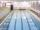Calculate slope (rise:run) of the bottom of swimming pool long 30 m. Water depth at beginning of pool is 1.13 m (for children) and depth at end is 1.84 m (for swimmers). Slope express as percentage and as angle in degrees.
4. Hexagonal prismThe base of the prism is a regular hexagon consisting of six triangles with side a = 12 cm and height va = 10.4 cm. The prism height is 5 cm. Calculate the volume and surface of the prism!
5. Shooter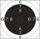The shooter fired to a target from distance 11 m The individual concentric circle of targets have a radius increments 1 cm (25 points) by 1 point. Shot was shifted by 8'(angle degree minutes). How many points should win his shot?
6. Nine-gonCalculate the perimeter of a regular nonagon (9-gon) inscribed in a circle with a radius 13 cm.
7. Sphere and coneWithin the sphere of radius G = 33 cm inscribe cone with largest volume. What is that volume and what are the dimensions of the cone?
8. Height differenceWhat height difference overcome if we pass road 1 km long with a pitch21 per mille?Average climb of the road is given by ratio 1:15. By what angle road average climb?
10. Elevation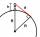What must be the elevation of an observer in order that he may be able to see an object on the earth 536 km away? Assume the earth to be a smooth sphere with radius 6378.1 km.
11. Triangle and its heightsCalculate the length of the sides of the triangle ABC, if va=5 cm, vb=7 cm and side b is 5 cm shorter than side a.
12. Euclid2In right triangle ABC with right angle at C is given side a=27 and height v=12. Calculate the perimeter of the triangle.
13. Sea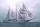How far can you see from the ship's mast, whose peak is at 14 meters above sea level? (Earth's radius is 6370 km).
14. Triangular prism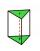The base perpendicular triangular prism is a right triangle whose hypotenuse measures 5 cm and one cathetus 2 cm. Height of the prism is equal to 7/9 of the perimeter of the base. Calculate the surface area of prism.
15. Triangular prismCalculate the surface area and volume of a triangular prism, base right triangle if a = 3 cm, b = 4 cm, c = 5 cm and height of prism h=12 cm.
16. The bridge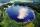Across the circle lakepasses through its center bridge over the lake. At three different locations on the lake shore are three fishermen A, B, C. Which of fishermen see the bridge under the largest angle?
17. Triangle KLBIt is given equilateral triangle ABC. From point L which is the midpoint of the side BC of the triangle it is drwn perpendicular to the side AB. Intersection of perpendicular and the side AB is point K. How many % of the area of the triangle ABC is area o
18. Cathethus and the inscribed circleIn a right triangle is given one cathethus long 14 cm and the radius of the inscribed circle of 5 cm. Calculate the area of this right triangle.
19. BoxCalculate the angle between box base 9 x 14 and body diagonal length 18.
20. Distance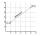Calculate distance between two points X[18; 19] and W[20; 3].

Do you have an interesting mathematical word problem that you can't solve it? Enter it, and we can try to solve it.

To this e-mail address, we will reply solution; solved examples are also published here. Please enter e-mail correctly and check whether you don't have a full mailbox.